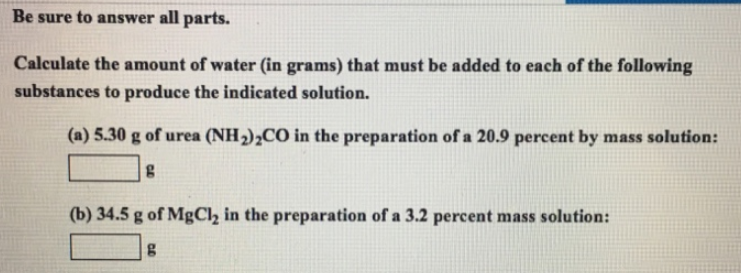# Problem: Be sure to answer all parts.Calculate the amount of water (in grams) that must be added to each of the following substances to produce the indicated solution. (a) 5.30 g of urea (NH2)2CO in the preparation of a 20.9 percent by mass solution: (b) 34.5 g of MgCl2 in the preparation of a 3.2 percent mass solution:

###### FREE Expert Solution
95% (427 ratings)###### Problem Details

Be sure to answer all parts.

Calculate the amount of water (in grams) that must be added to each of the following substances to produce the indicated solution.

(a) 5.30 g of urea (NH2)2CO in the preparation of a 20.9 percent by mass solution:

(b) 34.5 g of MgCl2 in the preparation of a 3.2 percent mass solution: